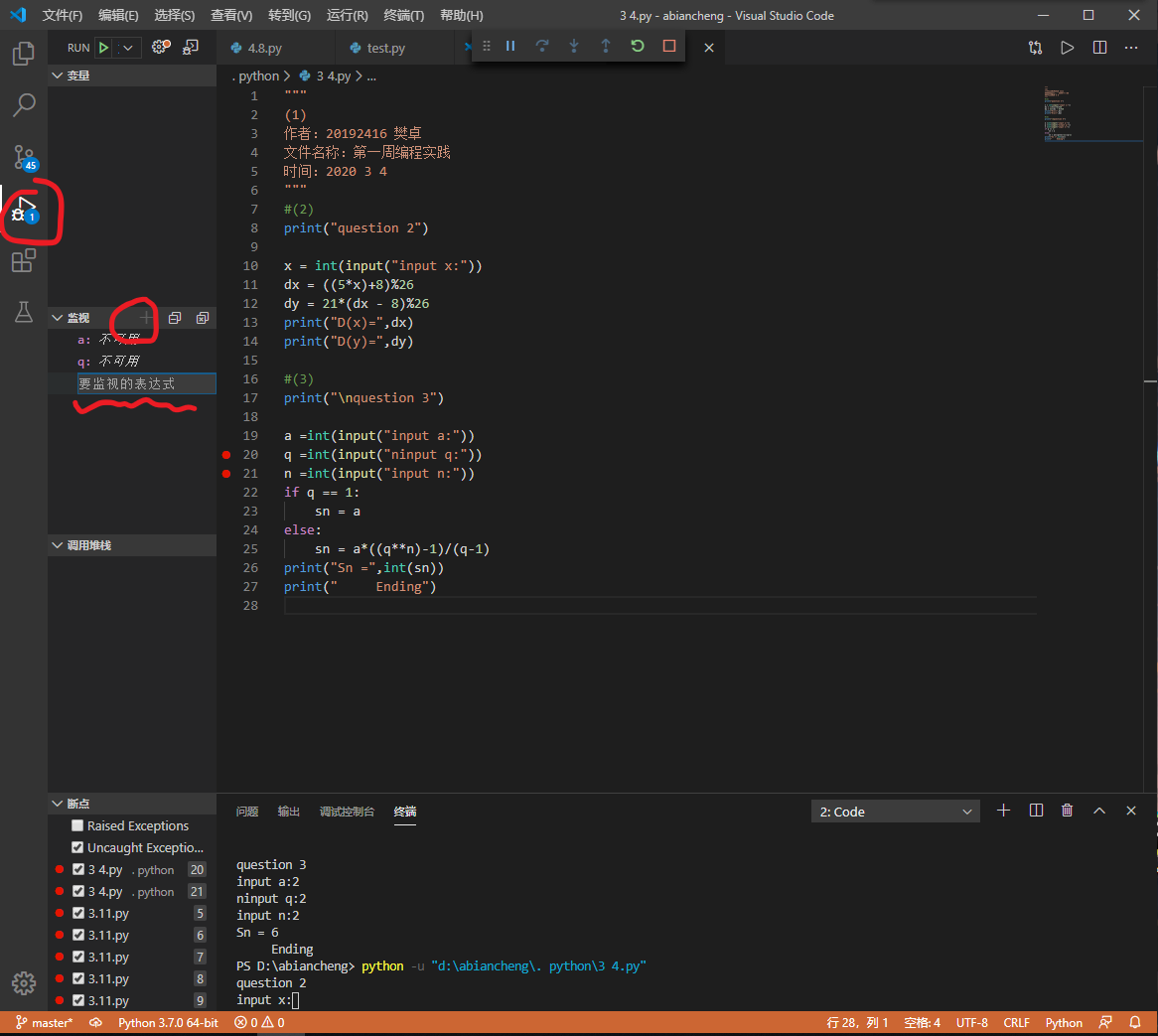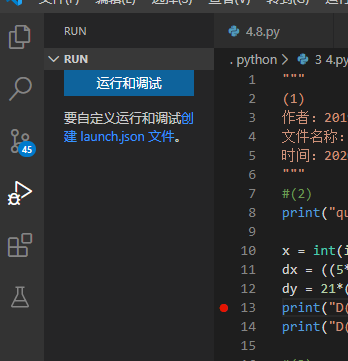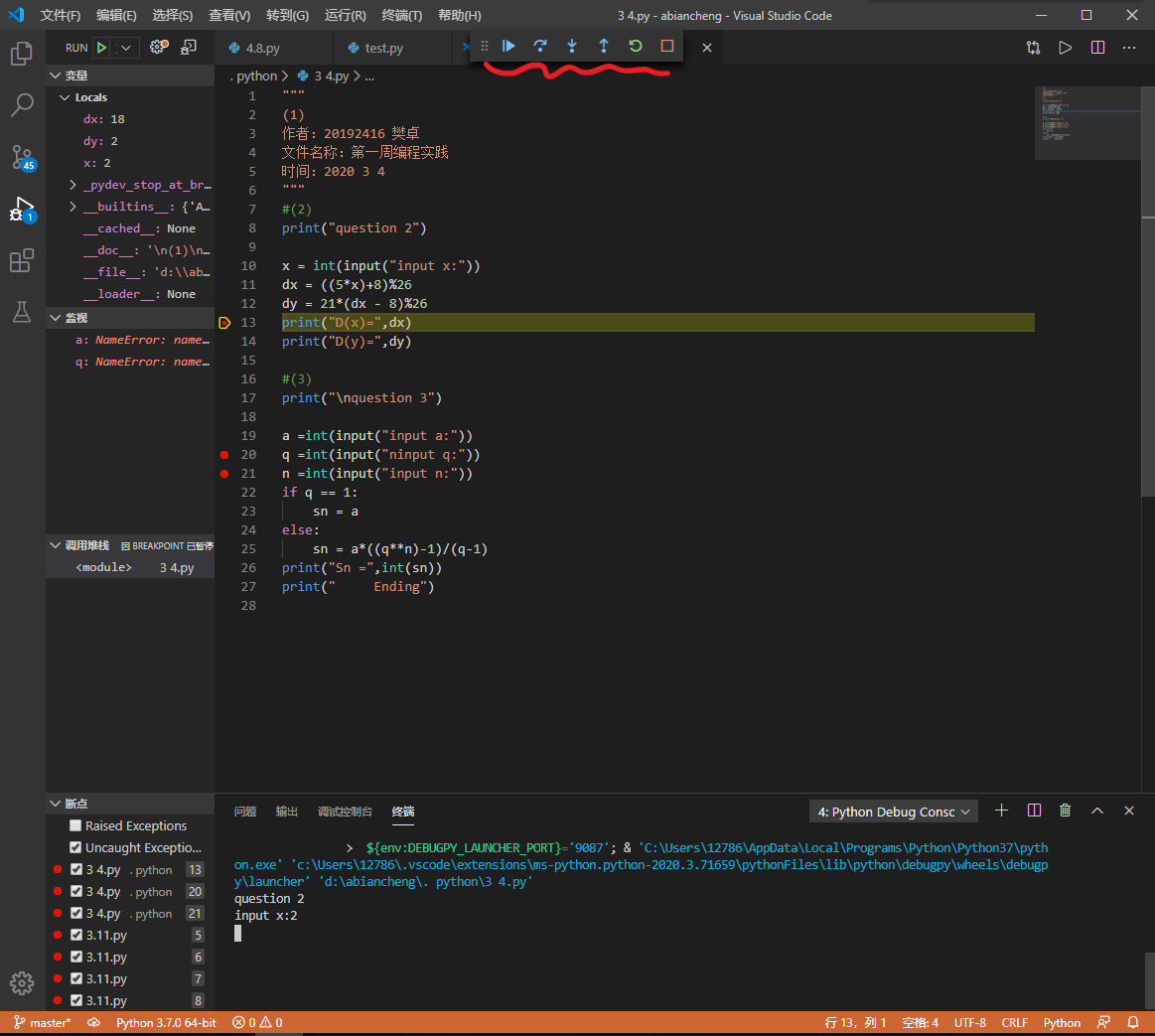# 20192416 2019-2020-2 《Python程序设计》实验一报告

## 1.实验内容

### （2）练习Python运行、调试技能；### （3）编写程序，练习变量和类型、字符串、对象、缩进和注释等；

python对缩进非常严格，缩进决定了他究竟在第几层。

1.直接在注释的内容前添加#。
2.大段内容注释可以在两头分别用两个'''括住。或选中内容，用ctrl+/将其注释掉。（快捷键真的非常方便）

### （4）掌握git技能

git commit -m "备注"
git push

## 2. 实验过程及结果

print("这是一个巨菜的计算器：")
a = int (input("输入0：结束    输入1：加   输入2：减   输入3：乘   输入4：除   "))
if a==1:
print("输入相加的数，最后一个数为0时结束")
b = int(input())
c=0
while(b!=0):
c=c+b
b = int(input())
print("他们的和为",c)
elif(a==2):
print("先输入被减数，依次输入减数，最后一个数为0时结束")
b = int(input())
c=b*2
while(b!=0):
c=c-b
b = int(input())
print("结果为",c)
elif a==3:
print("输入相乘的数，最后一个数为1时结束")
b = int(input())
c=1
while(b!=1):
c=c*b
b = int(input())
print("他们的积为",c)
elif a==4:
print("先输入被除数，依次输入除数，最后一个数为1时结束")
b = int(input())
c = b*b
while(b!=1):
c=c/b
b = int(input())
print("他们的结果为",c)
elif a==0:
print("结束")


## 3. 实验过程中遇到的问题和解决过程

• 问题1：输入不定个数的数据时，如何操作

• 问题1解决方案：
1.事先输入数据个数，决定循环次数。
2.输入到某个特殊数据时停止。
由于计算器的特殊性，最终决定用方法2。对于特殊数据，在加减运算中用0，在乘除运算中用1。

• 问题2：git代码存在困难

• 问题2解决方案：在网上到处找办法，一直不行。最终找到了官方《Git 仓库基础操作 》

## 其他（感悟、思考等）

python相对于C语言，更加贴近于人的思维。对于一些特殊问题，C语言许多行代码才能解决问题，python一行便可代替。
python的缩进非常严格，代码的缩进既是语言的规范，也增强了代码的可读性，具有一种独特的美感。

posted @ 2020-04-11 14:03  20192416  阅读(236)  评论(0编辑  收藏  举报